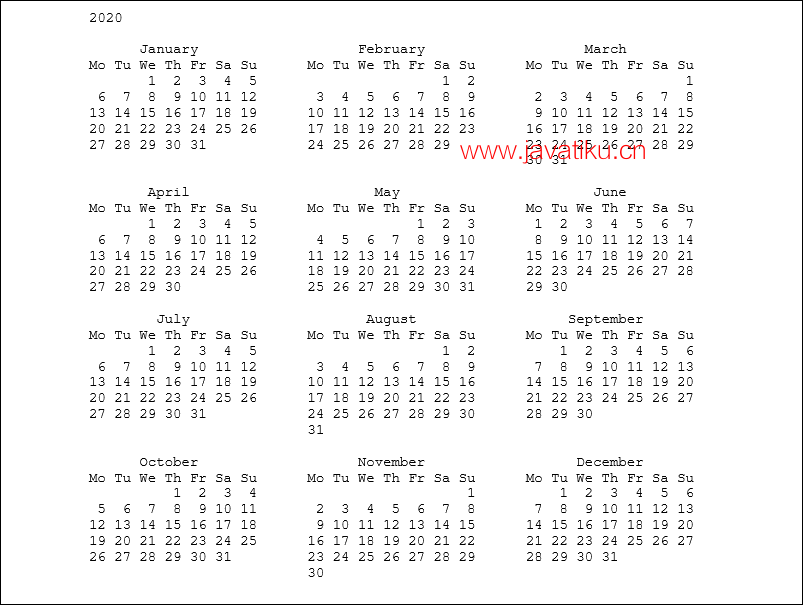# Tkinter 教程-Python 日期和时间Python 提供了 datetime 模块用于处理真实的日期和时间。在现实世界的应用中，我们需要处理日期和时间。Python 允许我们安排 Python 脚本在特定时间运行。

datetime 类被分为六个主要类别。

• date - 这是一个纯粹的理想日期。它包含年、月和日作为属性。
• time - 这是一个完美的时间，假设每天都精确地有 246060 秒。它有小时、分钟、秒、微秒和 tzinfo 作为属性。
• datetime - 这是日期和时间的组合，还包括年、月、日、小时、分钟、秒、微秒和 tzinfo 属性。
• timedelta - 它表示两个日期、时间或日期时间实例之间的差值，以微秒为分辨率。
• tzinfo - 它提供时区信息对象。
• timezone - 它包含在 Python 的新版本中。这是实现 tzinfo 抽象基类的类。

## 刻度（Tick）

``````import time;
#prints the number of ticks spent since 12 AM, 1st January 1970
print(time.time())  ``````

``1585928913.6519969``

## 如何获取当前时间？

``````import time;

#returns a time tuple

print(time.localtime(time.time()))  ``````

``time.struct_time(tm_year=2020, tm_mon=4, tm_mday=3, tm_hour=21, tm_min=21, tm_sec=40, tm_wday=4, tm_yday=94, tm_isdst=0)``

04 位数（例如 2018）
11 到 12
21 到 31
3小时0 到 23
4分钟0 到 59
50 到 60
6周的天数0 到 6
7年的天数1 到 366
8夏令时-1、0、1 或 -1

## 获取格式化的时间

``````import time
#returns the formatted time

print(time.asctime(time.localtime(time.time()))) ``````

``Tue Dec 18 15:31:39 2018``

## Python 的睡眠时间

time 模块的 sleep() 方法用于停止脚本的执行一段给定的时间。输出将会因为提供的秒数而被延迟。

``````import time
for i in range(0,5):
print(i)
#Each element will be printed after 1 second
time.sleep(1)  ``````

``````0
1
2
3
4``````

## datetime 模块

datetime 模块使我们能够创建自定义的日期对象，执行与日期相关的各种操作，如比较等。

``````import datetime
#returns the current datetime object
print(datetime.datetime.now())    ``````

``2020-04-04 13:18:35.252578``

## 创建日期对象

``````import datetime
#returns the datetime object for the specified date
print(datetime.datetime(2020,04,04))    ``````

``2020-04-04 00:00:00``

``````import datetime

#returns the datetime object for the specified time

print(datetime.datetime(2020,4,4,1,26,40))  ``````

``2020-04-04 01:26:40``

## 比较两个日期

``````from datetime import datetime as dt
#Compares the time. If the time is in between 8AM and 4PM, then it prints working hours otherwise it prints fun hours
if dt(dt.now().year,dt.now().month,dt.now().day,8)<dt.now()<dt(dt.now().year,dt.now().month,dt.now().day,16):
print("Working hours....")
else:
print("fun hours")   ``````

``fun hours``

## 日历模块

Python 提供了一个包含各种方法来处理日历的日历对象。

``````import calendar;
cal = calendar.month(2020,3)
#printing the calendar of December 2018
print(cal)    ``````

``````March 2020
Mo Tu We Th Fr Sa Su
1
2  3  4  5  6  7  8
9 10 11 12 13 14 15
16 17 18 19 20 21 22
23 24 25 26 27 28 29
30 31``````

## 打印整年的日历

``````import calendar
#printing the calendar of the year 2019
s = calendar.prcal(2020)  ``````